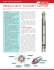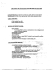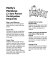# Analysis of power parameters of the squirrel cage motor and LSPMS

## Transcription

Analysis of power parameters of the squirrel cage motor and LSPMS
```Mariusz BARAŃSKI, Paweł IDZIAK, Wiesław ŁYSKAWIŃSKI, Wojciech SZELĄG
Poznan University of Technology, Institute of Electrical Engineering and Electronics
doi:10.15199/48.2015.04.30
Analysis of power parameters of the squirrel cage motor and
LSPMS motor with U-shaped magnets
Abstract. The paper presents the special algorithm and software for the transient FE analysis of electromagnetic phenomena in a squirrel cage
induction motor and in a line start permanent magnet synchronous motor. The presented algorithm and the elaborated specialized software for the
finite element analysis of transients and steady states are used to determine the power parameters of the squirrel-cage induction motor as well as
the Line Start Permanent Magnet Synchronous Motor (LSPMSM). The nonlinearity of the magnetic circuit, the movement of the rotor and the
skewed slots have been taken into account. The results of computations have been compared with measurements. The conclusions from the
comparison of calculation results with the results of measurements were presented. (Analiza parametrów energetycznych silnika indukcyjnego
klatkowego i silnika synchronicznego z magnesami w kształcie litery U o rozruchu bezpośrednim).
Streszczenie. W artykule przedstawiono model polowy oraz opracowane na jego podstawie algorytm oraz oprogramowanie do analizy procesu
rozruchu i ustalonych stanów pracy silnika indukcyjnego klatkowego oraz silnika synchronicznego magnetoelektrycznego przystosowanego do rozruchu
bezpośredniego. W rozważaniach uwzględniono nieliniowość obwodu magnetycznego, ruch wirnika oraz skoszenie żłobków wirnika w silniku
indukcyjnym. Obliczenia związane z uwzględnieniem skosu rdzenia stojana wykonano przy zastosowaniu modelu quasi-trójwymiarowego.
Zaprezentowano wybrane rezultaty badań symulacyjnych i laboratoryjnych. Przedstawiono wnioski wynikające z porównania wyników obliczeń
z rezultatami pomiarów.
Keywords: squirrel cage motor, LSPMS motor, finite element analysis, field-circuit model
Słowa kluczowe: silnik indukcyjny klatkowy, silnik synchroniczny magnetoelektryczny o rozruchu bezpośrednim, metoda elementów
skończonych, model polowo-obwodowy
Introduction
Induction motors still used in electric drives are being
increasingly replaced by synchronous motors with
permanent magnets (PMSM). This is due to the reduction of
operating costs of the latter ones, resulting from the
increase in the efficiency and power factor while reducing
their dimensions in comparison with conventional induction
motors of the same power. Many interesting PMSM
constructions have been described in the literature. Motors
with segmented magnets placed on the rotor surface are
presented in papers [10, 12]. In order to reduce time and
production costs, it is proposed e.g. to make motor
components of powder material in one technological
process [8, 14].
The main disadvantage of the PMSM is the necessity to
make use of electronic power converter systems to make
them start. This significantly increases the costs of the
entire drive system. In order to avoid this drawback,
intensive works on the LSPMSM are carried out in many
scientific research centers [7, 9, 11].
In this paper, the authors present the results of
simulation and laboratory tests of functional motor parameters, such as the efficiency, power factor and power output. A squirrel cage motor and the LSPMSM with the same
linear dimensions were examined. The calculations have
been performed with the use of computer software elaborated by the authors. The software has been prepared and
is successfully applied in the design of special squirrel cage
motors , e.g. motors working in cryogenic conditions .
Fig.1. The structures of rotors: a) IM, b) LSPMSM
In the design process of new constructions of the
LSPMSM, it is beneficial to utilize components of the classic
asynchronous motor. The key reason is the manufacturing
costs reduction, by keeping motor sizes and their interfaces
in the range of produced series of induction machines. The
components that can be easily taken from induction
machines (IM) are i.e. terminal boxes, stator cores,
housings, bearing and cooling systems. In order to reduce
the manufacturing costs of the LSPMS motor the prototype
of this synchronous motor has been based on the stator of
the considered squirrel-cage induction motor .
Examined motors
The Sg 100L-4B type, three-phase squirrel cage
induction motor with a power of PN=3 kW (Fig. 1a) and the
prototype of the designed LSPMSM motor (Fig.1b) were
examined. The same stator was used in both motors
(Fig.2b). The LSPMSM rotor included the starter squirrel
cage with 28 aluminum round rods and neodymium
magnets arranged in the U shape – Fig. 2.
Fig.2. Prototype of designed rotor of LSPMSM (a) and (c), stator of
the three phase induction motor, type Sg 100L-4B (b)
PRZEGLĄD ELEKTROTECHNICZNY, ISSN 0033-2097, R. 91 NR 4/2015
135
The use of the same stator core makes a comparative
analysis of energy parameters in both motors easier. In
order to achieve the optimal utilization of the magnetic
circuit the excitation system using a rare earth permanent
magnet has been designed . In the analysis of the
electromagnetic circuit of the motor, the influence of design
variables such as permanent magnet properties, cage
shape, magnet arrangement and the main dimensions of
the motor subcomponents on motor parameters have been
studied. The design and the optimization process have
been based on the circuit, field and field circuit models. To
avoid saturation of the stator it has been assumed that
amplitude of the first harmonic of the flux density in the airgap (excited by PM’s) should be close to the value
calculated for the base machine. The view of the designed
rotor prototype has been shown in Fig. 2c. In order to
reduce the costs of the prototype the small size stock
available NdFeB magnets have been utilized.
Finite element formulation
The mathematical model of electromagnetic phenomena
in a squirrel cage motor as well as a LSPMS motor include
the equations of the electromagnetic field and the
mechanical equilibrium equations of the considered
electromechanical system. In order to describe the
electromagnetic field the magnetic vector potential A and
the electric potential V have been applied . In this case,
the equation describing the transient electromagnetic field
in the motor can be expressed as .
(1)
(2a)
(2b)
1

     A   J  J m ,


J   dA dt  V  ,
Jm    M ,
(4)
B   H  ,
S nw  G w (1  C w )t 1

 z Tw

(7)
  nw 
 zw
  
 Rt  L   i n 
 M n  G (1  C w )t 1
  mw n    w
 z Tw
 tU  

0   n-1
w
  n 1 
 L  i 
,
where: n denotes the number of time-steps; w is the number
of rotor disk (sections) along the z axis parallel to the shaft
(for the LSPMSM w is equal 1); ∆t is the time-step length;
Mmw is the vector of magnetomotive force in regions with the
permanent magnets (for IM elements of matrix Mmw are equal
n
d
u  Ri  Ψ ,
dt
where u is the vector of supply voltages, i is the vector of
loop currents, R is the matrix of loop resistances, Ψ is the
flux linkage vector. The vector Ψ is calculated by means of
the field model.
The equations of field-circuit model are coupled through
the electromagnetic torque T to the equation of motion
136
where J is the moment of inertia,  is the angular position
of the rotor, TL is the load torque, Tf is the resistive torque
produced in the motor bearings and fan.
The electromagnetic torque T in equation (6) is calculated
on the basis of the magnetic field distribution .
In order to solve non-linear equations (1) – (6) only
approximate methods can be applied, based on the
discretization of space and time .
In the paper, the problem was considered as a twodimensional one, and so-called field-circuit methods were
used in the analysis of squirrel cage and LSPMS motors .
In order to represent the skew of the rotor slots, the
machine was cut into w disks (sections) along the z-axis
parallel to the shaft . Each section concerns the different
position of the rotor with respect to the stator. The magnetic
coupling of the disks is neglected. The stator winding of the
machine is composed of coils with thin conductors
connected in series. In the rotor, the squirrel-cage winding
is used. In our model, the windings are segmented into
elementary conductors associated with disks. The electric
connections of the stator and rotor windings sections are
taken into account. They are represented by circuit
equations . Moreover the resistances and inductances of
the stator winding end-turns and the rotor end-rings are
considered. Detailed description of the multi-slice model of
the rotor was presented in the papers [1, 2].
The time discretization leads to the following system of nonlinear algebraic matrix equations
to 0), S w , Gw, Cw, zw are the coefficients submatrices for
B  0 H  M  ,
where B is the vector of magnetic flux density, H is the
magnetic field strength vector,  0 is the magnetic
permeability of the vacuum.
In general, the transient electromagnetic field in the
electrical machines is voltage-excited. This means that the
phase currents in the windings are not known in advance,
i.e., prior to electromagnetic field calculation . Therefore,
it is necessary to consider the equations of the electric
circuit of the device. The set of independent loop equations
may be written as:
(5)
d 2
 TL  T f  T ,
dt 2
 
where  is the magnetic permeability, J is the current
density vector and σ is the conductivity of the medium, Jm
is the current density vector representing the magnetization
of the permanent magnet, M is the magnetization vector in
the region with permanent magnets.
In our considerations, it has been assumed that the
magnetic properties of magnetic soft materials and
permanent magnets are described by equation (3) and (4)
respectively.
(3)
J
(6)
disks; L is the matrix of end-turns inductances.
In order to approximate the time-derivatives in equation
(6) describing the motion of the rotors, an explicit formula
has been used
(8)
J αn 1  2αn  αn-1 / Δt   T  TL  T f .
The angular velocity
following the formula
2

of the rotor is determined by
 t n  0.5t    n 1   n  / t .
The movement of the rotor is simulated by means of the
moving band method. In the elaborated algorithm and
computer software equations (7) and (8) are solved with the
aid of the Newton-Raphson iterative procedure. The
electromagnetic torque was calculated using the FE
representation of the Maxwell stress tensor formula. The
formula was obtained by the analysis of the virtual
displacement corresponding to the moving band method.
(9)
PRZEGLĄD ELEKTROTECHNICZNY, ISSN 0033-2097, R. 91 NR 4/2015
Results
On the basis of the presented algorithm for solving
equations within the field-circuit model, the computer
program for the simulation of electromagnetic phenomena
in the full-scale model of squirrel-cage motor and the
LSPMS motor was elaborated. This program has been
developed in the Borland Delphi environment. It allows
automatic generation of mesh of the area with the user
selected software parameters such as: number of pole
pairs, the number of stator and rotor windings, single or
dual-layer winding, fractional slot winding, winding
connection. Developed procedures used in special
computer software allow you to choose different shapes of
motor slots. For the purpose of the program the base of
magnetic soft and hard materials has been developed. The
analysis of the phenomena in the considered motors can be
carried out without taking into account as well as
considering the skewed rotor slots. The program allows to
carry out the calculations using a three-phase motor power
system sinusoidal voltage as well as distorted voltages. The
results of the calculation can be visualized as graphs,
waveforms, electromagnetic field distribution, schedules
and later saved as a file for further processing.
On the basis of the calculations the efficiency, the power
factor and the line current as a function of the load torque
were determined for the examined motors. The calculation
results compared with the measurement results are listed in
Fig. 3-5. The obtained characteristics confirm the
improvement of the LSPMSM operating parameters (the
higher efficiency and power factor and lower current in
stator windings for the same load) in comparison with the
parameters of the induction motor with the same stator.
In order to compare the energy parameters of both motor
constructions, the values of efficiency η, power factor cosφ,
currents in stator winding I and power output Pu are
summarized in Table 1. For the rated load torque T = 20 Nm,
the current of the LSPMSM is by about 20% lower than that
of the induction motor. It results from the lower power loss
and makes possible to increase the load torque of the
LSPMSM to T = 24 Nm. As a result, an increase of the
power output of the LSPMSM by over 25 % was obtained in
comparison with that one of the induction motor. The power
output increase is due to both the load torque increase and
the rotational speed increase up to 1500 rpm.
Table 1. Selected functional parameters in steady state obtained
during laboratory tests
motor
IM
LSPMSM
parameters T=20Nm T=20Nm T=24Nm
η [%]
0.81
0.86
0.88
cosφ [-]
Fig.3. Efficiency as a function of load torque
0.81
0.93
0.94
I [A]
7.2
6.0
7.1
Pu [kW]
3.0
3.1
3.8
Conclusions
After application of the rotor with the properly shaped
magnetic circuit equipped with permanent magnets and the
starter squirrel cage in the induction motor, it is possible to
obtain a synchronous motor capable of self-starting, even
under load. The effective parameters of the LSPMSM are
better than those of the induction motor. The power factor
and the efficiency of the motor with permanent magnets are
significantly higher than those obtained from the induction
motor. The effective current value of the LSPMSM in
steady-state is also lower by approx. 20 % than that of the
induction motor for the same load of both machines. The
results of simulation tests and measurements confirm that it
would be advisable to implement this type of motor for the
production and use. The usefulness of the software
developed for the computer aided design of the cage
induction motor and the LSPMS motor have been proven.
REFERENCES
Fig.4. Power factor as a function of load torque
Fig.5. Current as a function of load torque
 B a r a ń s k i M . , D e m e n k o A . , Ł y s k a w i ń s k i W . , S z e l ą g
W ., Finite element analysis of transient electromagneticthermal phenomena in a squirrel cage motor, COMPEL, 30
(2011), No. 3, 832 – 840
 B a r a ń s k i . M . , S z e l ą g W . , Finite element analysis of
transient electromagnetic-thermal phenomena in a squirrel
cage motor working at cryogenic temperature, IET Science
Measurement and Technology, 6 (2012), No 5, 357 – 363
 B a r a ń s k i
M.,
Szeląg
W.,
Jędryczka
C.,
M i k o ł a j e w i c z J . , Ł u k a s z e w i c z P . , Analiza i badanie
silnika synchronicznego o rozruchu bezpośrednim i magnesach
w wirniku rozłożonych w kształcie litery U. Przegląd
Elektrotechniczny, R. 89, Nr 2b/2013, 107-111
 D e m e n k o A . , Movement simulation in finite element analysis
of electric machine dynamics”, IEEE Transactions on
Magnetics, 32 (1996), No 3, 1553-1556
 D r i e s e n J . , Coupled electromagnetic-thermal problems in
electrical energy transducers, (2000), Katholieke Universiteit
Leuven.
PRZEGLĄD ELEKTROTECHNICZNY, ISSN 0033-2097, R. 91 NR 4/2015
137
 D r i e s e n J . , B e l m a n s R . J . M . , H a m e y e r K . ,
Methodologies for Coupled Transient Electromagnetic-Thermal
Finite-Element Modeling of Electrical Energy Transducers,
IEEE Transactions on Industry Applications, 38 (2004), No 5,
1244-1250
 F e i . W . , L u k K . P . C . , M a J . , S h e n J . X . , Y a n g G . , A
high-performance line-start permanent magnet synchronous
motor amended from a small industrial three-phase induction
motor, IEEE Transactions on Magnetics, 45 (2009), No. 1,
4724-4727
 G w o ź d z i e w i c z M . , Z a w i l a k J . , Influence of the rotor
construction on the single-phase line start permanent magnet
synchronous motor performances, Przegląd Elektrotechniczny,
R.87, NR 11/2011, 135-138
 K n i g h t A . M . , M c C l a y C . I , The design of high-efficiency
line-start motors, IEEE Transactions on Industry Applications,
36 (2000), No. 6, 1555 -1562
 M a y H . , P a l k a R . , P a p l i c k i P . , S z k o l n y S . ,
C a n d e r s W . - R . , Modified concept of permanent magnet
excite synchronous machines with improved high-speed
features, Archives of Electrical Engineering,
60 (2011),
No. 4, 531-540
 M i l l e r T . J . E . , P o p e s c u M . , C o s s a r C . , M c G i l p
M.I., Strappazzon G., Trivillin N., Santarossa R.,
Line start permanent magnet motor: single-phase steady-state
performance analysis, IEEE Transactions on Industry
Applications, 40 (2004), No. 2, 516-525
 M ł o t A . , K o r k o s z M . , Ł u k a n i s z y n M . , Iron loss and
eddy-current loss analysis in a low-power BLDC motor with
138
magnet segmentation, Archives of Electrical Engineering, 61
(2012), No. 1, 33-46
 S z e l ą g
W.,
Finite
element
analysis
of
the
magnetorheological fluid brake transients, Compel, 23 (2004),
No. 6, 758-766
 W o j c i e c h o w s k i R . M . , J ę d r y c z k a C . , Ł u k a s z e w i c z
P . , K a p e l s k i D . , Analysis of high speed permanent magnet
motor with powder material, COMPEL, 31 (2012), No. 5, 15281540
Autorzy:
dr inż. Mariusz Barański, Politechnika Poznańska, Instytut
Elektrotechniki i Elektroniki Przemysłowej, ul. Piotrowo 3a, 60-965
Poznań, E-mail: [email protected]
dr hab. inż. Paweł Idziak, Politechnika Poznańska, Instytut
Elektrotechniki i Elektroniki Przemysłowej, ul. Piotrowo 3a, 60-965
Poznań, E-mail: [email protected]
dr hab. inż. Wiesław Łyskawiński, Politechnika Poznańska, Instytut
Elektrotechniki i Elektroniki Przemysłowej, ul. Piotrowo 3a, 60-965
Poznań, E-mail: [email protected]
prof. dr hab. inż. Wojciech Szeląg, Politechnika Poznańska, Instytut
Elektrotechniki i Elektroniki Przemysłowej, ul. Piotrowo 3a, 60-965
Poznań, E-mail: [email protected]
e-mail: [email protected]
PRZEGLĄD ELEKTROTECHNICZNY, ISSN 0033-2097, R. 91 NR 4/2015
```

### Crumble Maker Cards Motor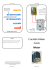### T-REX Manual - RC Helitechnics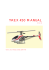### Answering the following questions correctly will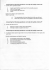### COMMERCIAL BOOTHS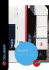### Work and Power Practice Problems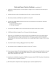### Hamilton Beach 936 3 Speed Milk Shake / Malt Mixer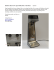### Pump- Set Features: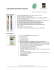### Permanent Magnet Motor